# 渲染球体

## 视口>>>>>>>>>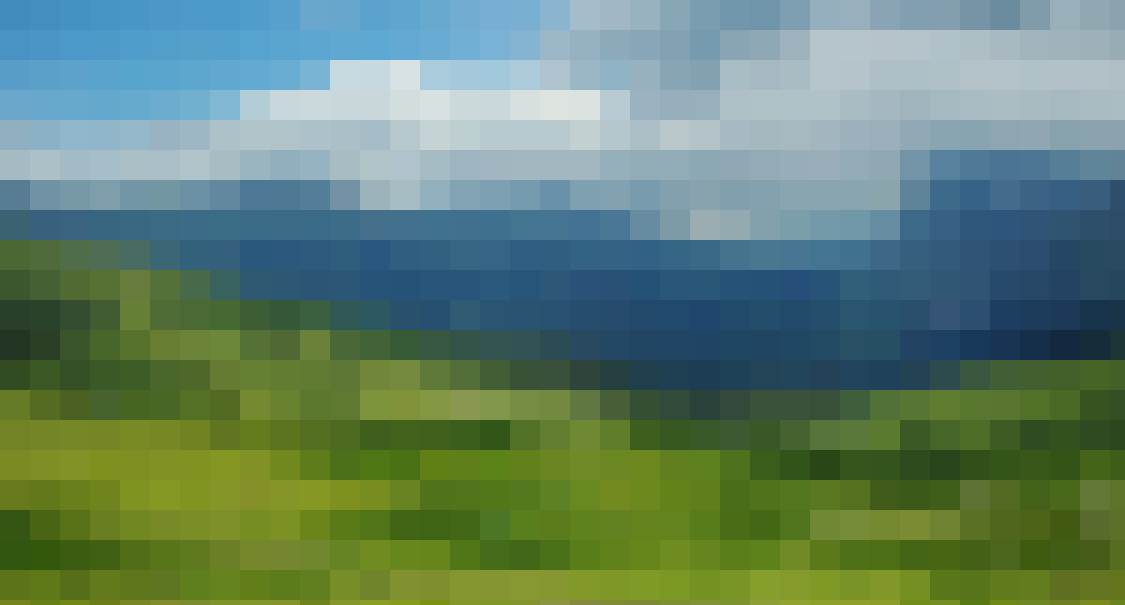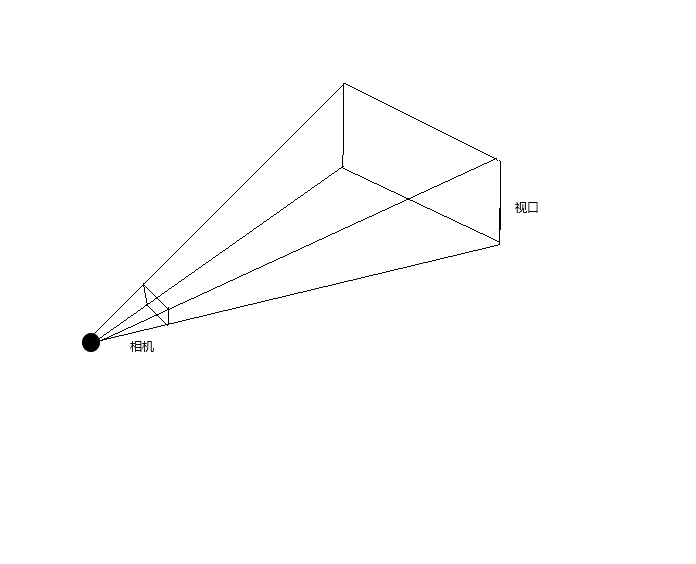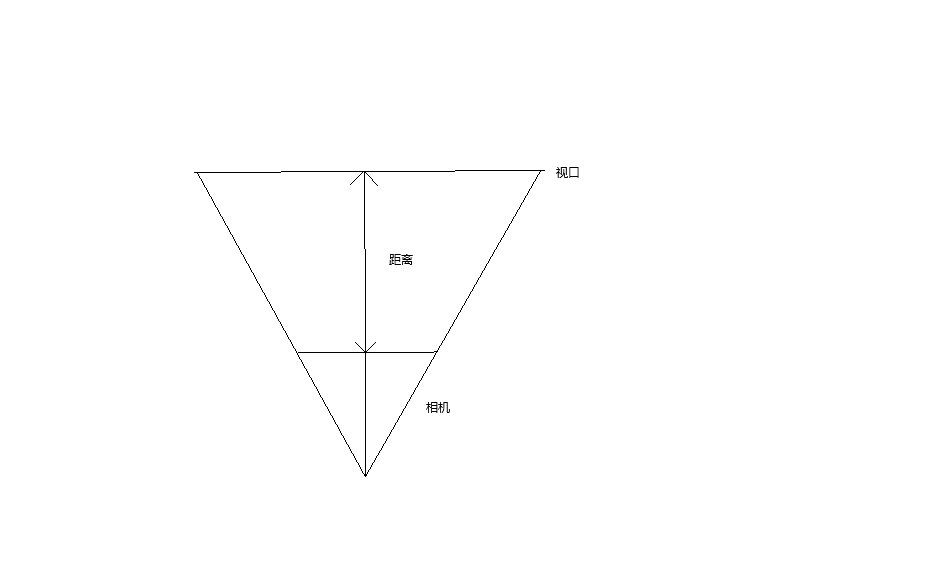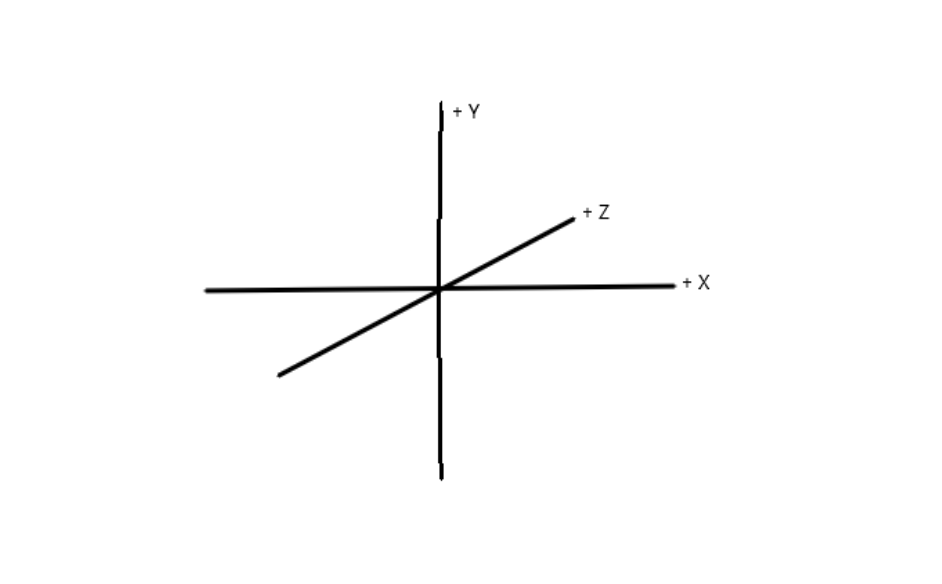Vx=Vw/Cw*Cx

Vy=Vh/Ch*Cy

## 追踪射线

O是原点，D是（空间内的某一点的坐标V，V-O），t是前进的数量。tD就是个向量数乘，D可以理解为方向。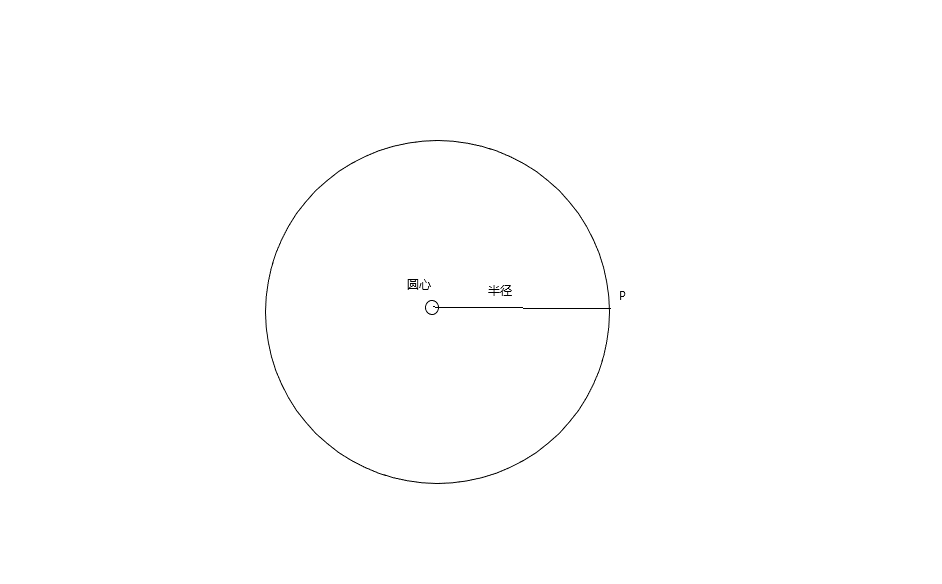r2 =(P-C ) dot (P-C)

r2 =根号下（P-C）的点积。

(O+tD-C)dot(O+tD-C)=r2 ，我们利用交换律替换成（O-C+tD）,O-C是一个向量，我可以用OC代替。最终是

(OC+tD) dot (OC+tD)=r2 ,因为是点积，我们可以利用分配律展开第一个括号

OC dot（OC+tD）+ tD dot (OC+tD)=r即 为：

OC dot OC+ OC dot tD+ tD dot OC +tD dot tD=r2

(OC dot OC)+2(OC Dot tD)+(tD dot tD)=r2,因为点乘可以引用结合律，所以

2OC dot tD=2(t(OC dot D))=2t(OC dot D)

(tD dot tD)=t*(D) dot t*(D)=t*(D dot t*D)=t*(t*(D dot D ))=t^2(D dot D)

(OC dot OC)+2t(OC dot D)+t^2(D dot D)=r^2。

a=(D dot D)

b=2(OC dot D)

c =(OC dot OC)-r^2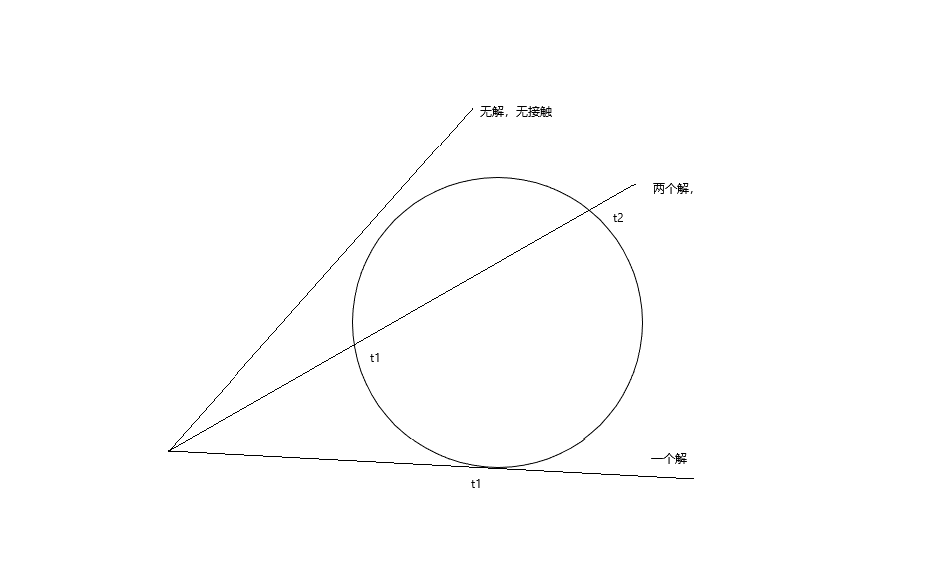## 代码

　　　　class sharp
{
public Vector3D center
{
get; set;
}
public double radius { get; set; } = 1;
public Color color
{
get; set;
}
}

 　　　　  List<sharp> sharplist = new List<sharp>
{
new sharp() { center = new Vector3D(0, -1, 3), color = Colors.Red },
new sharp() { center = new Vector3D(2, 0, 4),  color = Colors.Blue },
new sharp() { center = new Vector3D(-2, 0, 4), color = Colors.Green }
};

　　　　 /// <summary>
/// 求解一元二次
/// </summary>
/// <param name="origin">起点</param>
/// <param name="dline">向量</param>
/// <param name="sharp">球体</param>
/// <returns></returns>
Point IntersectRayShere(Vector3D origin, Vector3D dline, sharp sharp)
{
//半径
//起点到圆心
var co = origin - sharp.center;

var a = Vector3D.DotProduct(dline, dline);

var b = 2 * Vector3D.DotProduct(co, dline);

var c = Vector3D.DotProduct(co, co) - r * r;

var discrinimant = b * b - 4 * a * c;

if (discrinimant < 0)
{
return new Point();
}
var t1 = (-b + Math.Sqrt(discrinimant)) / (2 * a);
var t2 = (-b - Math.Sqrt(discrinimant)) / (2 * a);
//偷懒
return new Point(t1, t2);
}

光线追踪

    /// <summary>
/// 光线追踪
/// </summary>
/// <param name="origin">起点</param>
/// <param name="dline">方向</param>
/// <param name="min">射线最小值</param>
/// <param name="max">射线最大值</param>
Color tracrray(Vector3D origin, Vector3D dline, double min, double max)
{
double closet = double.PositiveInfinity;
sharp claset_sharp = null;
//遍历模型
foreach (var item in sharplist)
{
//求解是否与圆相交
var gp = IntersectRayShere(origin, dline, item);
//小于判断
if (gp.X >= min && gp.X <= max && gp.X < closet)
{
closet = gp.X;
claset_sharp = item;
}
if (gp.Y >= min && gp.Y <= max && gp.Y < closet)
{
closet = gp.Y;
claset_sharp = item;
}
}
//不存在返回空白值
if (claset_sharp == null)
{
return Colors.White;
}
//
return claset_sharp.color;
}

  　　　　　　WriteableBitmap.Lock();
//画布开始
for (double x = 0 - cw / 2; x < cw / 2; x++)
{
for (double y = 0 - ch / 2; y < ch / 2; y++)
{
//画布到视口
var D = canvastoviewport(new Point(x, y));
//获取像素颜色
var color = tracrray(new Vector3D(), D, 1, double.PositiveInfinity);
//坐标系转换
var p = MidPoint(new Point(x, y));
//定义颜色BGRA
byte[] colorData = { color.B, color.G, color.R, color.A };
//跨距
int stride = (WriteableBitmap.PixelWidth * WriteableBitmap.Format.BitsPerPixel) / 8;
//绘制
WriteableBitmap.WritePixels(new Int32Rect((int)p.X, (int)p.Y, 1, 1), colorData, stride, 0);
}
}
WriteableBitmap.Unlock();

于是我们得到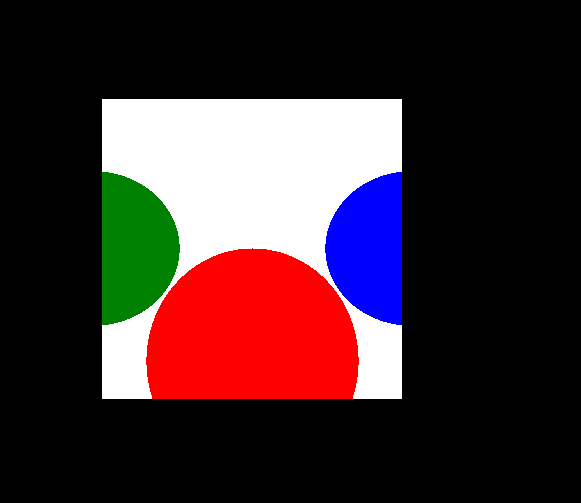posted @ 2022-06-24 14:23  ARM830  阅读(66)  评论(1编辑  收藏  举报